Part 5 | IFT World
IFT Notes for Level I CFA® Program
IFT Notes for Level I CFA® Program

# Part 5

## 8. Quantiles

### 8.1 Quartiles, Quintiles, Deciles, and Percentiles

A quantile is a value at or below which a stated fraction of the data lies. Some examples of quantiles include:

• Quartiles: The distribution is divided into quarters.
• Quintiles: The distribution is divided into fifths.
• Deciles: The distribution is divided into tenths.
• Percentile: The distribution is divided into hundredths.

The formula for the position of a percentile in a data set with n observations sorted in ascending order is: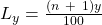where:
y is the percentage point at which we are dividing the distribution.
n is the number of observations.
Ly is the location (L) of the percentile (Py) in an array sorted in ascending order.

Some important points to remember are:

• When the location, Ly, is a whole number, the location corresponds to an actual observation.
• When Ly is not a whole number or integer, Ly lies between the two closest integer numbers (one above and one below) and we use linear interpolation between those two places to determine Py.
• Interquartile range is the difference between the third and the first quartiles.

Example

Consider the data set:

47        35        37        32        40        39        36        34        35        31       44

1. Find the 75th percentile point
2. Find the 1st quartile and 3rd quartile
3. Calculate the interquartile range
4. Find the 5th decile point
5. Find the 6th decile point.

Solution to 1:

First arrange the data in ascending order:

31, 32, 34, 35, 35, 36, 37, 39, 40, 44, 47

Location of the 75th percentile is the:
L75 = (11 + 1) (75/100) = 9th value. i.e. P75 = 40

With a small data set, such as this one, the location and the value is approximate. As the data set becomes larger, the location and percentile value estimates become more precise.

Solution to 2:

Location of the 1st quartile is:

L25 = (11 + 1) (25/100) = 3rd value. i.e. P25 = 34

Location of the 3rd quartile is:

L75 = (11 + 1) (75/100) = 9th value. i.e. P75 = 40

Solution to 3:

The interquartile range is the difference between the third and first quartiles, 40 – 34 = 6

Solution to 4:

Location of the 5th decile is:

L50 = (11 + 1) (50/100) = 6th value. i.e. P50 = 36

Solution to 5:

L60 = (11 + 1) (60/100) = 7.2

Use linear interpolation, which estimates an unknown value on the basis of two known values that surround it.

In this case, the 7th value is 37 and the 8th value is 39. The 6th decile is: P60 = 37+ 0.4 (0.2 times the linear distance between 37 and 39). P60 = 37.4

A box and whiskers plot is used to visualize the dispersion of data across quartiles. The box represents the interquartile range. The whiskers represent the highest and lowest values of the distribution. Exhibit 44 shows a sample box and whisker plot.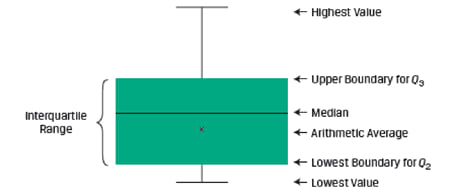There are several variations of the box and whiskers plot. Sometimes the whiskers may be a function of the interquartile range instead of the highest and lowest values.

### 8.2 Quantiles in Investment Practice

Quantiles are used in:

• Portfolio performance evaluation: The performance of investment managers is often evaluated in terms of the percentile or quartile in which they fall relative to the performance of their peers.
• Investment research: For example, companies can be ranked based on their market capitalization and sorted into deciles. The first decile contains companies with smallest market values and the tenth decile contains companies with the largest market values. Such a classification allows analysts to compare the performance of small companies with large ones.

## 9. Measures of Dispersion

Measures of central tendency tell us where the investment results (expected returns) are centered. However, to evaluate an investment we also need to know how returns are dispersed around the mean. Measures of dispersion describe the variability of outcomes around the mean.

### 9.1 The Range

The range is the difference between the maximum and minimum values in a data set. It is expressed as:

Range = Max value – Min Value

If the annual returns data is: 10%, -5%, 10%, 25%. What is the range?

Here the maximum return is 25% and the minimum return is -5%. The range is 25% – (-5%) = 30%.

Another way to specify the range is to mention the actual minimum and maximum values. For example, for the above data the range is “from -5% to 25%”.

The range is easy to compute; however, it does not tell us much about how the data is distributed.

### 9.2 The Mean Absolute Deviation

It is the average of the absolute values of deviations from the mean. It is expressed as:

MAD=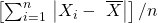where: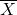is the sample mean and n is the number of observations in the sample.

Example

Consider the following data set: 8, 12, 10, 8 and 5. Calculate the mean absolute deviation.

Solution:= (8 + 12 + 10 + 8 + 5) / 5 = 8.6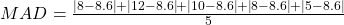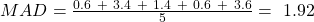### 9.3 Sample Variance and Sample Standard Deviation

Variance is defined as the average of the squared deviations around the mean. Standard deviation is the positive square root of the variance.

Sample variance applies when we are dealing with a subset, or sample, of the total population. It is expressed as: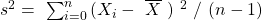where:is the sample mean and n is the number of observations in the sample.

Sample standard deviation is defined as the positive square root of the sample variance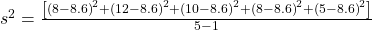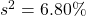The sample standard deviation is the positive square root of the sample variance. For the sample data given above, s= √6.80 = 2.61%

Using a financial calculator to calculate variance and standard deviations

The sample standard deviation can easily be computed using a financial calculator. Assume the following data set: 10%, -5%, 10%, 25%, the calculator key strokes are shown below:

 Keystrokes Description Display [2nd] [DATA] Enters data entry mode [2nd] [CLR WRK] Clears data register X01 10 [ENTER] X01 = 10 [↓] [↓] 5+/- [ENTER] X02 = -5 [↓] [↓] 10 [ENTER] X03 = 10 [↓] [↓] 25 [ENTER] X04 = 25 [2nd] [STAT] [ENTER] Puts calculator into stats mode [2nd] [SET] Press repeatedly till you see 🡪 1-V [↓] Number of data points N = 4 [↓] Mean X = 10 [↓] Sample standard deviation Sx = 12.25 [↓] Population standard deviation σx = 10.61

Notice that the calculator gives both the sample and the population standard deviation.  On the exam we will have to determine whether we are dealing with population or sample data and choose the appropriate value.

Dispersion and the relationship between the arithmetic and the geometric means

The sample standard deviation can be used to understand the gap between the arithmetic and geometric mean. The relationship between the arithmetic mean () and geometric mean(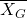) is: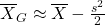The larger the variance of the sample, the wider the difference between the geometric mean and the arithmetic mean.

Example:

The dividend yield for five hypothetical companies from a list of ten companies is given below. What is the sample variance?

 Paknama 10.50% Genie Ltd. 16.25% Mirinda Corp. 27.00% Tina Travels Ltd. 12.00% Thomas Press Ltd. 7.80%

Solution: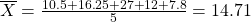Sample variance=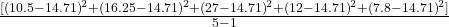Sample variance = 56.49

## 10. Downside Deviation and Coefficient of Variation

Variance and standard deviation of returns take account of returns above and below the mean, but often investors are concerned only with downside risk, for example returns below the mean.

The target downside deviation, or target semideviation, is a measure of the risk of being below a given target. It is calculated as the square root of the average squared deviations from the target, but it includes only those observations below the target (B).

The sample target semideivation can be calculated as: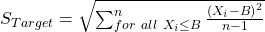Example:

Suppose the monthly returns on a portfolio are as shown:

 Month Return (%) Jan 6 Feb 4 Mar -2 Apr -5 May 5 Jun 2 Jul 1 Aug 0 Sep 4 Oct 3 Nov 0 Dec 2

Calculate the target downside deviation when the target return is 4%.

Solution:

 Month Observation Deviation from the 4% target Deviation below the target Squared deviations below the target Jan 6 2 – – Feb 4 0 – – Mar -2 -6 -6 36 Apr -5 -9 -9 81 May 5 1 – – Jun 2 -2 -2 4 Jul 1 -3 -3 9 Aug 0 -4 -4 16 Sep 4 0 – – Oct 3 -1 -1 1 Nov 0 -4 -4 16 Dec 2 -2 -2 4 Sum 167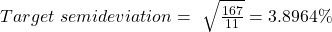The target downside deviation will be less than the standard deviation, because deviations above the target are ignored. As the target is increased, the target downside deviation will increase.

### 10.1 Coefficient of Variation

Coefficient of variation expresses how much dispersion exists relative to the mean of a distribution and allows for direct comparison of dispersion across different data sets, even if the means are drastically different from one another. It is used in investment analysis to compare relative risks. When evaluating investments, a lower value is better. Coefficient of variation is expressed as: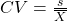where: s = sample standard deviation of a set of observations and= sample mean

Example

Investment A has a mean return of 7% and a standard deviation of 5%. Investment B has a mean return of 12% and a standard deviation of 7%. Calculate the coefficients of variation.

Solution

The coefficients of variation can be calculated as follows: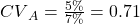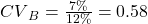This metric shows that Investment A is riskier than Investment B.